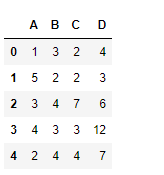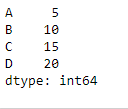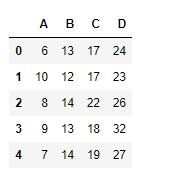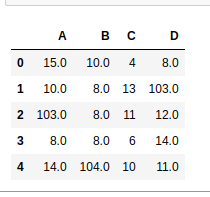Open in App
Not now

• Last Updated : 27 Aug, 2021

Python is a great language for doing data analysis, primarily because of the fantastic ecosystem of data-centric python packages. Pandas is one of those packages and makes importing and analyzing data much easier.
Pandas dataframe.radd() function performs the addition of the dataframe and other object element-wise. Other object could be a constant, series or a dataframe. The function essentially performs other + dataframe but with an additional support of fill_value, which fills all the missing value in one of the inputs.
For series input, the index must match.
Note : This is different from dataframe.add() function. As in this function we add the dataframe to the other object.

Parameters :
other : Series, DataFrame, or constant
axis : For Series input, axis to match Series index on
level : Broadcast across a level, matching Index values on the passed MultiIndex level
fill_value : Fill existing missing (NaN) values, and any new element needed for successful DataFrame alignment, with this value before computation. If data in both corresponding DataFrame locations is missing the result will be missing
Returns : result : DataFrame

Example #1: Use radd() function to perform addition of a dataframe with a series

## Python3

 `# importing pandas as pd``import` `pandas as pd` `# Creating the dataframe``df ``=` `pd.DataFrame({``"A"``:[``1``, ``5``, ``3``, ``4``, ``2``],``                   ``"B"``:[``3``, ``2``, ``4``, ``3``, ``4``],``                   ``"C"``:[``2``, ``2``, ``7``, ``3``, ``4``],``                   ``"D"``:[``4``, ``3``, ``6``, ``12``, ``7``]})` `# Print the dataframe``df`Let’s create a series with matching index as that of the dataframe column axis

## Python3

 `# importing pandas as pd``import` `pandas as pd` `# Create a Series``sr ``=` `pd.Series([``5``, ``10``, ``15``, ``20``], index ``=``[``"A"``, ``"B"``, ``"C"``, ``"D"``])` `# Print the series``sr`## Python3

 `# add dataframe to the series over the column axis``df.radd(sr, axis ``=` `1``)`

Output :## Python3

 `# importing pandas as pd``import` `pandas as pd` `# Creating the first dataframe``df1 ``=` `pd.DataFrame({``"A"``:[``1``, ``5``, ``3``, ``4``, ``2``],``                    ``"B"``:[``3``, ``2``, ``4``, ``3``, ``4``],``                    ``"C"``:[``2``, ``2``, ``7``, ``3``, ``4``],``                    ``"D"``:[``4``, ``3``, ``6``, ``12``, ``7``]})` `# Creating the second dataframe``df2 ``=` `pd.DataFrame({``"A"``:[``14``, ``5``, ``None``, ``4``, ``12``],``                    ``"B"``:[``7``, ``6``, ``4``, ``5``, ``None``],``                    ``"C"``:[``2``, ``11``, ``4``, ``3``, ``6``],``                    ``"D"``:[``4``, ``None``, ``6``, ``2``, ``4``]})` `# add two dataframes``df1.radd(df2, fill_value ``=` `100``)`

Output :My Personal Notes arrow_drop_up